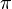# Variable facts for kids

Kids Encyclopedia Facts

A variable is a special type of amount or quantity with an unknown value. The opposite of a variable (that is, a known value) is called a constant.

## Variables in math

In mathematics, a variable is usually given a letter, such as x or y. For example:

• The letters m, n, p, q are often used as variables for integers.
• The letters a, b and c are often used as coefficients of functions.
• The letters i, j ,k are often used as indices of summation.
• The letter t is often used as a variable for time.
• The letter z is often used as a variable for complex numbers.

Most letters are used in equations to show numbers that are unknown (an exception is e, which is a constant). Using a type of math called algebra, one can find the value of the variable.

Any number cannot change its value. This is true whether the number is rational (such as -8.625) or irrational (such as 2√3, which is 2 multiplied by the square root of 3), or whether the number is real (such as$\pi$) or imaginary (such as$i$).

Variables are also common in science, where they usually represent physical properties that do not stay the same. For example, if a person stands 5 feet away from a building, the distance variable compared to the building does not change over time. 5 feet is called a fixed amount, which is the opposite of a variable amount. But if a person walks away from the building, the distance variable compared to the building is increasing. So it does not have the same value and we cannot use the same number for it, as the distance might be 1 foot now, but 2 feet a second later.

## Variables in computer science

In computer science, a variable is a value in a program that can change. It does not have to be a number. In fact, it can be a string (text value), a date, an amount of money, an object such as a picture, or simply null (which means it has no content). The value that is stored in a variable can change the behavior of a program when it is run. Because of this, variables are commonly used to store input and output values.

Each programming language works differently with variables. Most of them allow any character string that is not "reserved" (that is, has a special meaning) to be the name of the variable. It is good in computer programming to use names that tells what will be stored in the variable (such as "person", "age", "total", and so on). Also, some languages like C and Java require the data type of the variable (that is, the kind of content that will be stored in it) to be declared (written) when the variable is created. Others, like Python and Visual Basic, do not have this rule, which allows the type of variable to be changed when a new value is stored in it.

## Related pagesVariable Facts for Kids. Kiddle Encyclopedia.